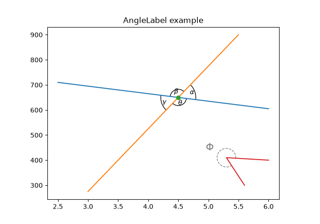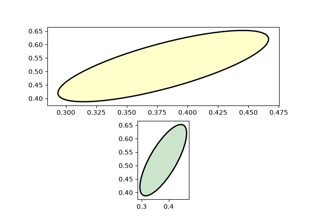# matplotlib.patches.Arc#

class matplotlib.patches.Arc(xy, width, height, *, angle=0.0, theta1=0.0, theta2=360.0, **kwargs)[source]#

Bases: `Ellipse`

An elliptical arc, i.e. a segment of an ellipse.

Due to internal optimizations, the arc cannot be filled.

Parameters:
xy(float, float)

The center of the ellipse.

widthfloat

The length of the horizontal axis.

heightfloat

The length of the vertical axis.

anglefloat

Rotation of the ellipse in degrees (counterclockwise).

theta1, theta2float, default: 0, 360

Starting and ending angles of the arc in degrees. These values are relative to angle, e.g. if angle = 45 and theta1 = 90 the absolute starting angle is 135. Default theta1 = 0, theta2 = 360, i.e. a complete ellipse. The arc is drawn in the counterclockwise direction. Angles greater than or equal to 360, or smaller than 0, are represented by an equivalent angle in the range [0, 360), by taking the input value mod 360.

Other Parameters:
**kwargs`Patch` properties

Most `Patch` properties are supported as keyword arguments, except fill and facecolor because filling is not supported.

Property

Description

`agg_filter`

a filter function, which takes a (m, n, 3) float array and a dpi value, and returns a (m, n, 3) array and two offsets from the bottom left corner of the image

`alpha`

unknown

`animated`

bool

bool or None

`capstyle`

`CapStyle` or {'butt', 'projecting', 'round'}

`clip_box`

`Bbox`

`clip_on`

bool

`clip_path`

Patch or (Path, Transform) or None

`color`

color

`edgecolor` or ec

color or None

`facecolor` or fc

color or None

`figure`

`Figure`

`fill`

bool

`gid`

str

`hatch`

{'/', '\', '|', '-', '+', 'x', 'o', 'O', '.', '*'}

`in_layout`

bool

`joinstyle`

`JoinStyle` or {'miter', 'round', 'bevel'}

`label`

object

`linestyle` or ls

{'-', '--', '-.', ':', '', (offset, on-off-seq), ...}

`linewidth` or lw

float or None

`mouseover`

bool

`path_effects`

`AbstractPathEffect`

`picker`

None or bool or float or callable

`rasterized`

bool

`sketch_params`

(scale: float, length: float, randomness: float)

`snap`

bool or None

`transform`

`Transform`

`url`

str

`visible`

bool

`zorder`

float

draw(renderer)[source]#

Draw the arc to the given renderer.

Notes

Ellipses are normally drawn using an approximation that uses eight cubic Bezier splines. The error of this approximation is 1.89818e-6, according to this unverified source:

Lancaster, Don. Approximating a Circle or an Ellipse Using Four Bezier Cubic Splines.

https://www.tinaja.com/glib/ellipse4.pdf

There is a use case where very large ellipses must be drawn with very high accuracy, and it is too expensive to render the entire ellipse with enough segments (either splines or line segments). Therefore, in the case where either radius of the ellipse is large enough that the error of the spline approximation will be visible (greater than one pixel offset from the ideal), a different technique is used.

In that case, only the visible parts of the ellipse are drawn, with each visible arc using a fixed number of spline segments (8). The algorithm proceeds as follows:

1. The points where the ellipse intersects the axes (or figure) bounding box are located. (This is done by performing an inverse transformation on the bbox such that it is relative to the unit circle -- this makes the intersection calculation much easier than doing rotated ellipse intersection directly.)

This uses the "line intersecting a circle" algorithm from:

Vince, John. Geometry for Computer Graphics: Formulae, Examples & Proofs. London: Springer-Verlag, 2005.

2. The angles of each of the intersection points are calculated.

3. Proceeding counterclockwise starting in the positive x-direction, each of the visible arc-segments between the pairs of vertices are drawn using the Bezier arc approximation technique implemented in `Path.arc`.

set(*, agg_filter=<UNSET>, alpha=<UNSET>, angle=<UNSET>, animated=<UNSET>, antialiased=<UNSET>, capstyle=<UNSET>, center=<UNSET>, clip_box=<UNSET>, clip_on=<UNSET>, clip_path=<UNSET>, color=<UNSET>, edgecolor=<UNSET>, facecolor=<UNSET>, fill=<UNSET>, gid=<UNSET>, hatch=<UNSET>, height=<UNSET>, in_layout=<UNSET>, joinstyle=<UNSET>, label=<UNSET>, linestyle=<UNSET>, linewidth=<UNSET>, mouseover=<UNSET>, path_effects=<UNSET>, picker=<UNSET>, rasterized=<UNSET>, sketch_params=<UNSET>, snap=<UNSET>, transform=<UNSET>, url=<UNSET>, visible=<UNSET>, width=<UNSET>, zorder=<UNSET>)[source]#

Set multiple properties at once.

Supported properties are

Property

Description

`agg_filter`

a filter function, which takes a (m, n, 3) float array and a dpi value, and returns a (m, n, 3) array and two offsets from the bottom left corner of the image

`alpha`

scalar or None

`angle`

float

`animated`

bool

bool or None

`capstyle`

`CapStyle` or {'butt', 'projecting', 'round'}

`center`

(float, float)

`clip_box`

`Bbox`

`clip_on`

bool

`clip_path`

Patch or (Path, Transform) or None

`color`

color

`edgecolor` or ec

color or None

`facecolor` or fc

color or None

`figure`

`Figure`

`fill`

bool

`gid`

str

`hatch`

{'/', '\', '|', '-', '+', 'x', 'o', 'O', '.', '*'}

`height`

float

`in_layout`

bool

`joinstyle`

`JoinStyle` or {'miter', 'round', 'bevel'}

`label`

object

`linestyle` or ls

{'-', '--', '-.', ':', '', (offset, on-off-seq), ...}

`linewidth` or lw

float or None

`mouseover`

bool

`path_effects`

`AbstractPathEffect`

`picker`

None or bool or float or callable

`rasterized`

bool

`sketch_params`

(scale: float, length: float, randomness: float)

`snap`

bool or None

`transform`

`Transform`

`url`

str

`visible`

bool

`width`

float

`zorder`

float

## Examples using `matplotlib.patches.Arc`#Scale invariant angle label

Scale invariant angle labelEllipse with units

Ellipse with units